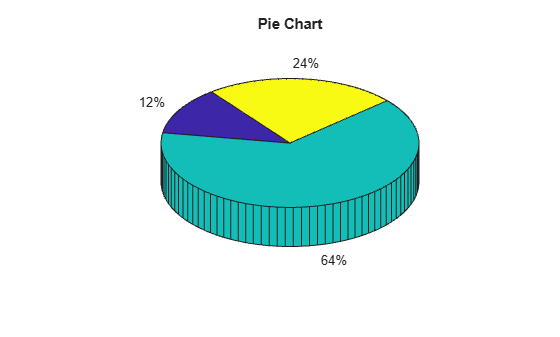# switch, case, otherwise

Execute one of several groups of statements

## Syntax

```switch `switch_expression`
case `case_expression`
`statements`
case `case_expression`
`statements`
...
otherwise
`statements`
end
```

## Description

example

``````switch switch_expression, case case_expression, end``` evaluates an expression and chooses to execute one of several groups of statements. Each choice is a case. The `switch` block tests each case until one of the case expressions is true. A case is true when:For numbers, `case_expression == switch_expression`.For character vectors, ```strcmp(case_expression,switch_expression) == 1```.For objects that support the `eq` function, ```case_expression == switch_expression```. The output of the overloaded `eq` function must be either a logical value or convertible to a logical value.For a cell array `case_expression`, at least one of the elements of the cell array matches `switch_expression`, as defined above for numbers, character vectors, and objects.When a case expression is true, MATLAB® executes the corresponding statements and exits the `switch` block.An evaluated `switch_expression` must be a scalar or character vector. An evaluated `case_expression` must be a scalar, a character vector, or a cell array of scalars or character vectors.The `otherwise` block is optional. MATLAB executes the statements only when no case is true.```

## Examples

collapse all

Display different text conditionally, depending on a value entered at the command prompt.

```n = input('Enter a number: '); switch n case -1 disp('negative one') case 0 disp('zero') case 1 disp('positive one') otherwise disp('other value') end```

At the command prompt, enter the number 1.

`positive one`

Repeat the code and enter the number 3.

`other value`

Determine which type of plot to create based on the value of `plottype`. If `plottype` is either `'pie'` or `'pie3'`, create a 3-D pie chart. Use a cell array to contain both values.

```x = [12 64 24]; plottype = 'pie3'; switch plottype case 'bar' bar(x) title('Bar Graph') case {'pie','pie3'} pie3(x) title('Pie Chart') otherwise warning('Unexpected plot type. No plot created.') end```## Tips

• A `case_expression` cannot include relational operators such as `<` or `>` for comparison against the `switch_expression`. To test for inequality, use `if, elseif, else` statements.

• The MATLAB `switch` statement does not fall through like a C language `switch` statement. If the first `case` statement is `true`, MATLAB does not execute the other `case` statements. For example:

```result = 52; switch(result) case 52 disp('result is 52') case {52, 78} disp('result is 52 or 78') end```
`result is 52`
• Define all variables necessary for code in a particular case within that case. Since MATLAB executes only one case of any `switch` statement, variables defined within one case are not available for other cases. For example, if your current workspace does not contain a variable `x`, only cases that define `x` can use it:

```switch choice case 1 x = -pi:0.01:pi; case 2 % does not know anything about x end```
• The MATLAB `break` statement ends execution of a `for` or `while` loop, but does not end execution of a `switch` statement. This behavior is different than the behavior of `break` and `switch` in C.

## Version History

Introduced before R2006a